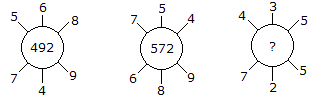# Verbal Reasoning - Character Puzzles - Discussion

### Discussion :: Character Puzzles - Character Puzzles 1 (Q.No.6)

6.

Which one will replace the question mark ?[A]. 115 [B]. 130 [C]. 135 [D]. 140

Explanation:

(5 x 6 x 8) + (7 x 4 x 9) = 492

and (7 x 5 x 4) + (6 x 8 x 9) = 572

Therefore (4 x 3 x 5) + (7 x 2 x 5) = 130.

 Santhosh said: (Sep 19, 2011) I didn't understand the puzzle, can any one explain me it in detail?

 Kani said: (Dec 25, 2015) Its very simple as shown in explanation.

 Ganesh said: (Aug 1, 2016) It very simple to understand.

 Yamini said: (Aug 20, 2016) @Santhosh. It's very simple, please read the explanation once again, You will get it.

 Manaswee Singh said: (Mar 25, 2019) 1 step - Multiply the upper portion 4,3and5 4*3*5=60. 2nd step-Multiply the lower portion 7,2and5 7*2*5=70. 3rd step- Now add the calculation of both 1st step and 2nd step (4*3*5) + (7*2*5), 60+70 = 130ans.

 Anil Kumar said: (Mar 21, 2020) Please explain briefly I can't understand this type of Puzzle.

 Yessmin said: (Sep 18, 2020) Thanks for explaining @Manaswee.

 Mouni said: (Dec 19, 2020) Thanks all for explaining.

 Anomie said: (Oct 25, 2021) Step 1. Multiply upper numbers by lower numbers; That is; ( 5 * 6 * 8 ) + ( 7 * 4 * 9 ) = 492 ( the middle number). Step 2; The same way the second one follows this rule. Step 3; ( 4 * 3 * 5 ) + (7 * 2 * 5 ), = 130, Therefore, 130 is the answer!